Open in App
Not now

# Class 8 RD Sharma Solutions – Chapter 3 Squares and Square Roots – Exercise 3.8

• Last Updated : 28 Dec, 2020

### i) 5

Solution: We will use Long Division Method to find square root of 5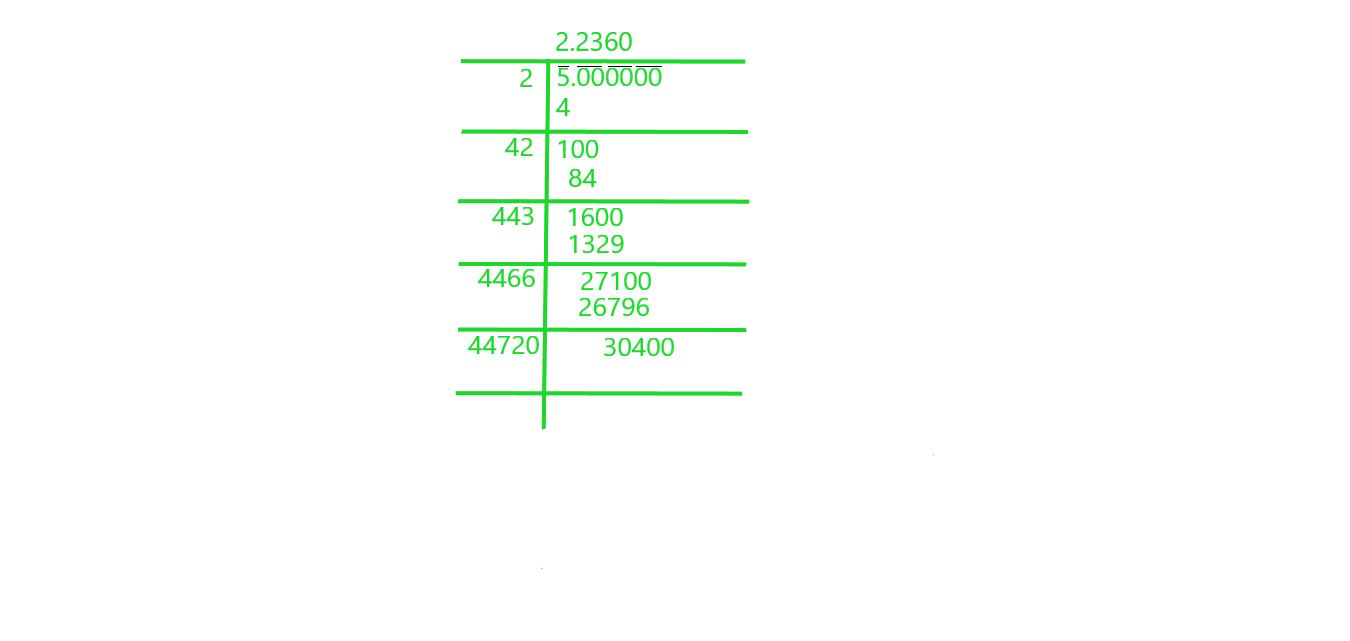Square root of 5 is 2.2360

### ii) 7

Solution: We will use Long Division Method to find square root of 7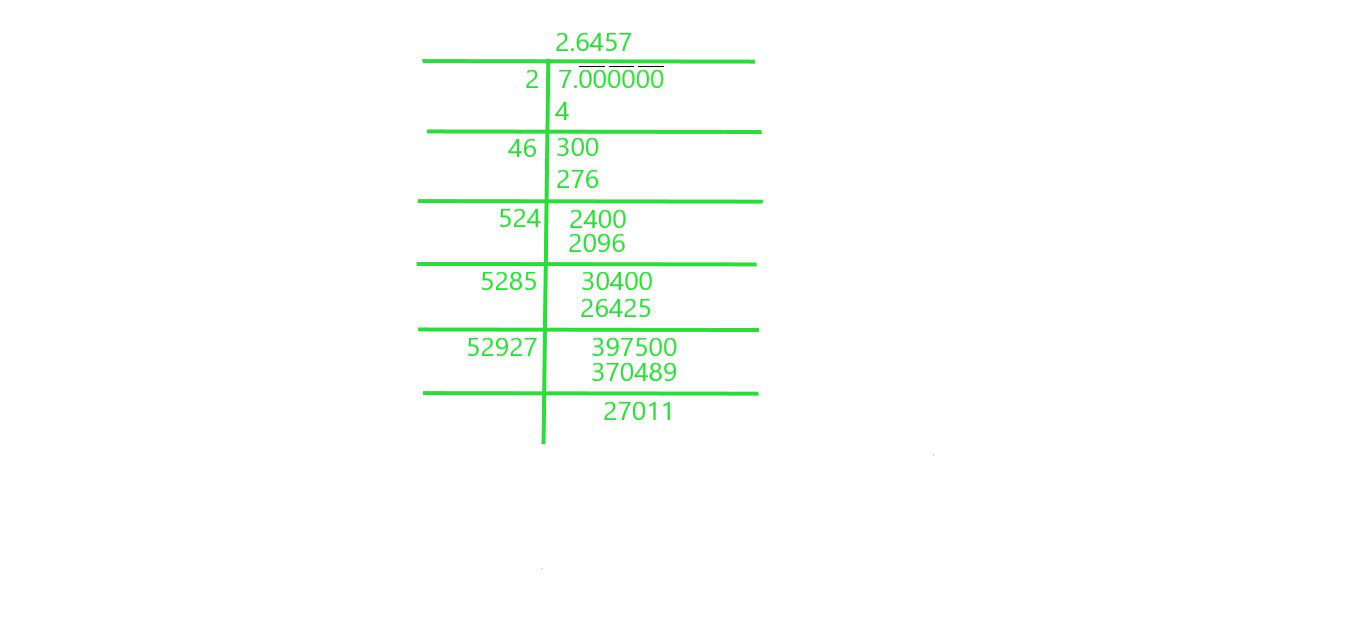Square root of 7 is 2.6457

### iii) 17

Solution: We will use Long Division Method to find square root of 17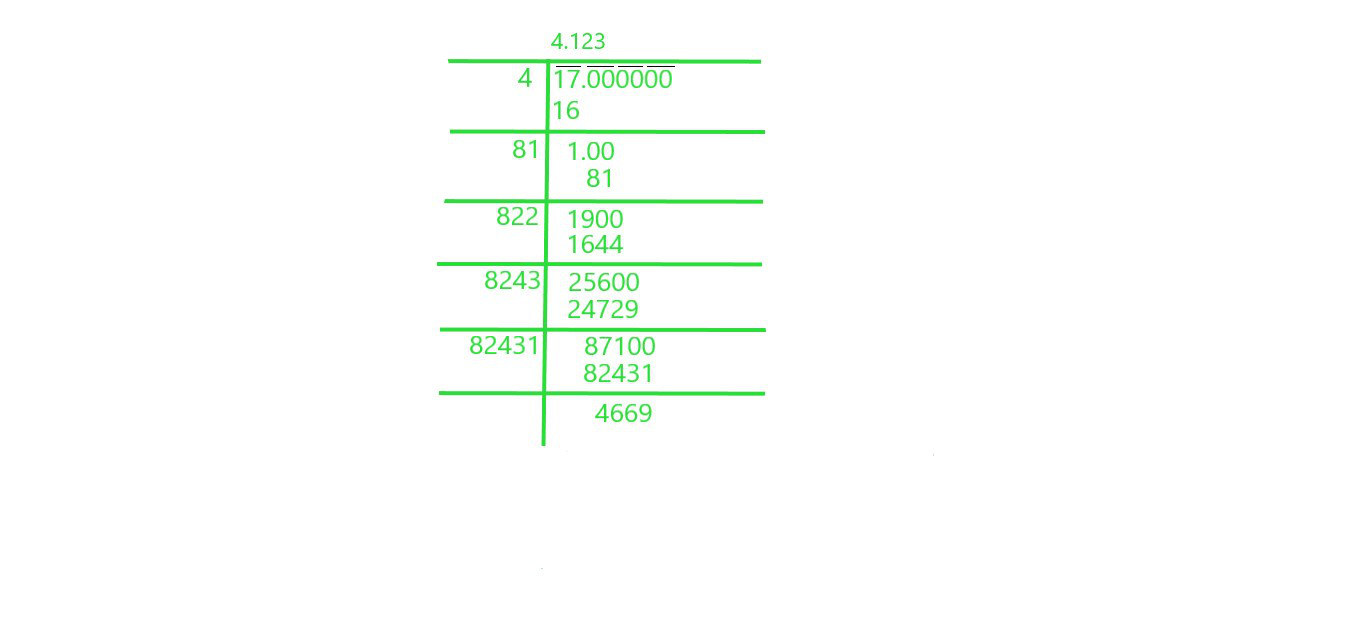Square root of 17 is 4.123

### iv) 20

Solution: We will use Long Division Method to find square root of 20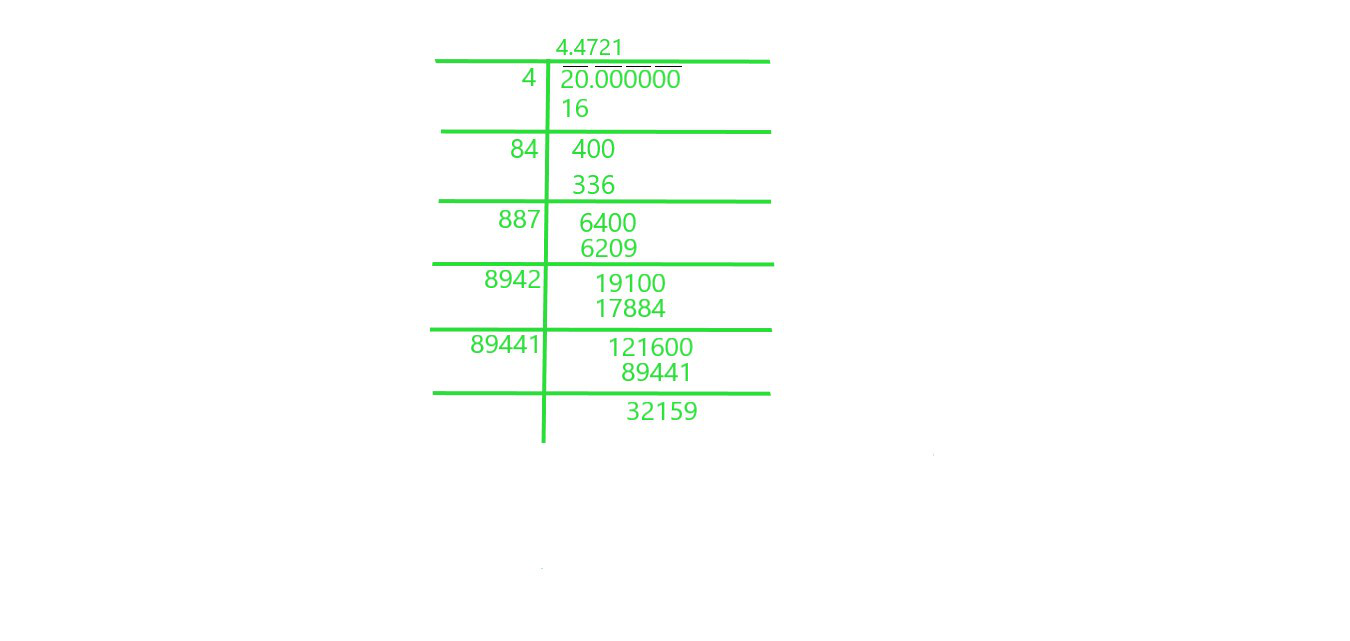Square root of 20 is 4.4721

### v) 66

Solution: We will use Long Division Method to find square root of 66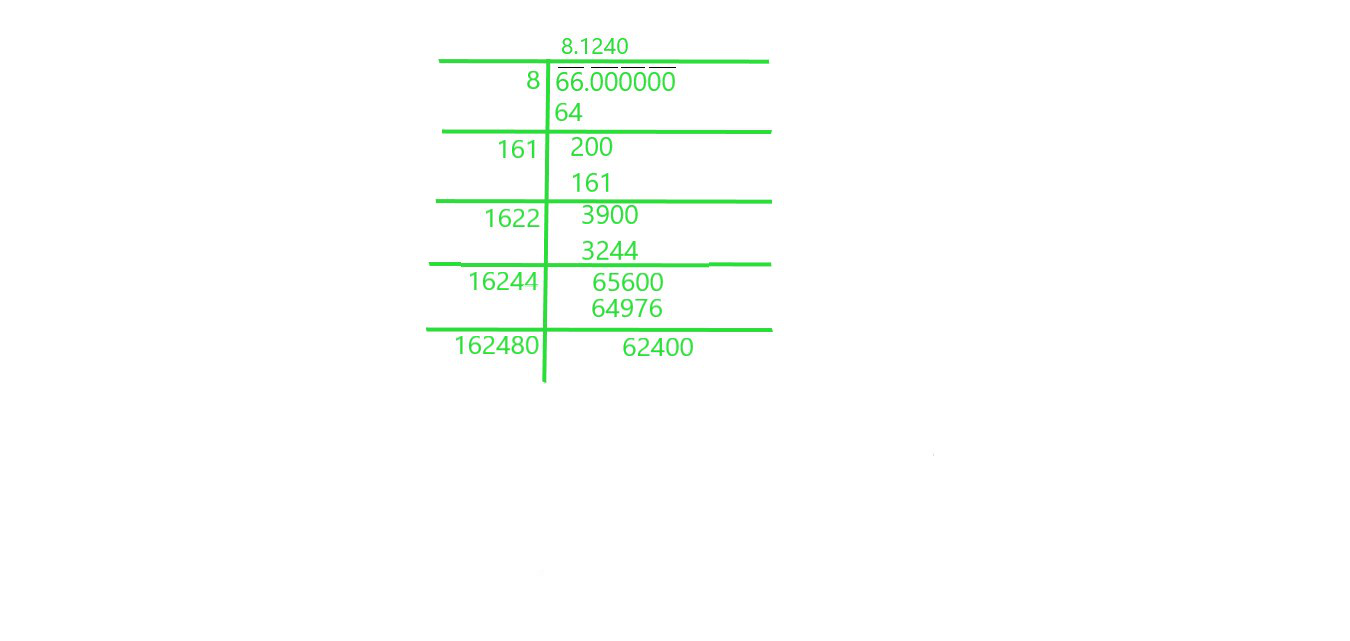Square root of 66 is 8.1240

### vi) 427

Solution: We will use Long Division Method to find square root 427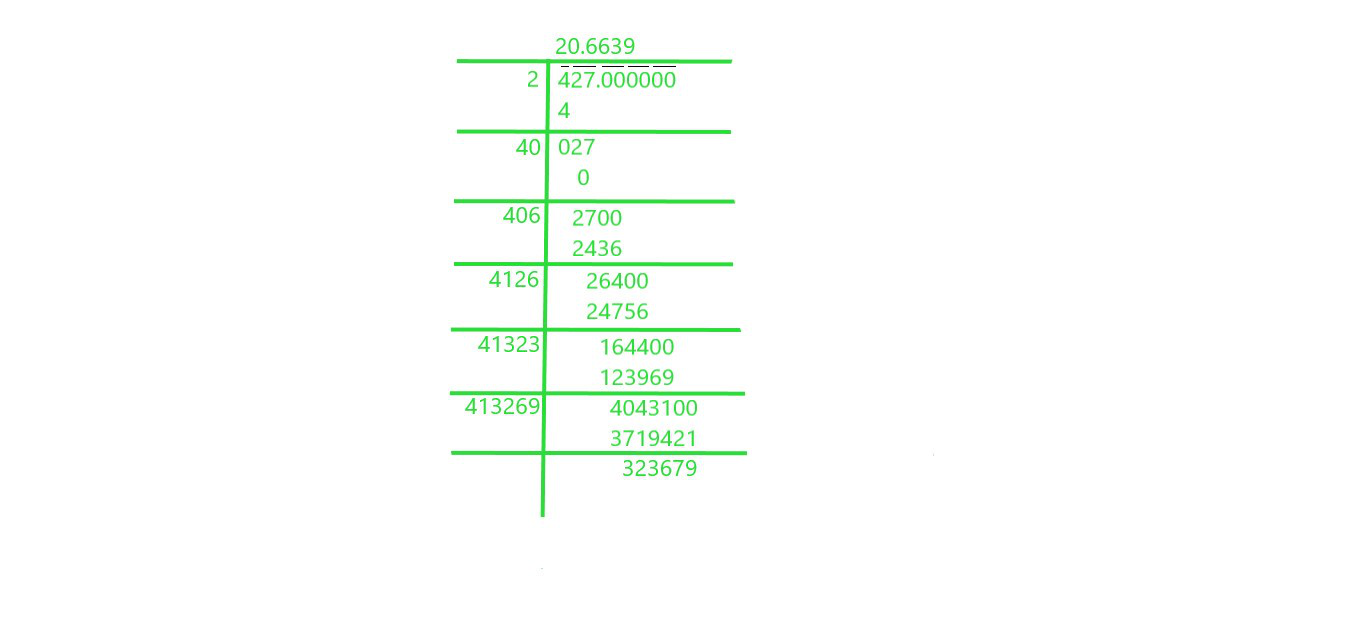Square root of 427 is 20.6639

### vii) 1.7

Solution: We will use Long Division Method to find square root of 1.7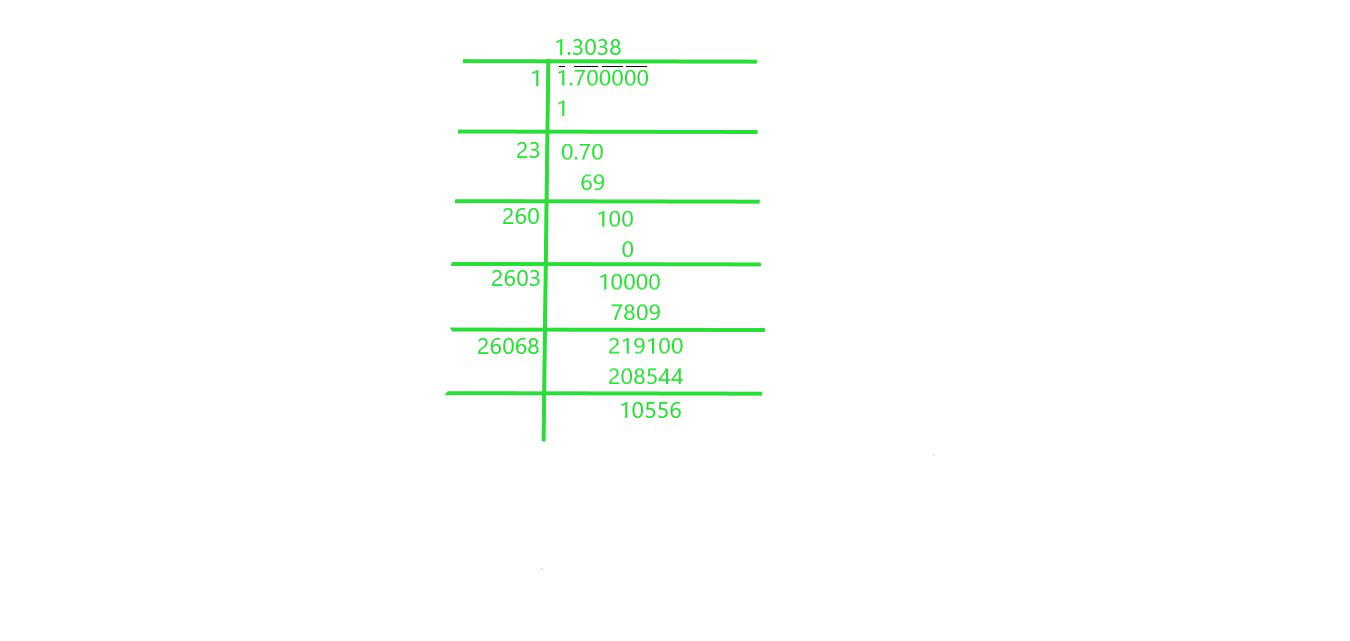Square root of 1.7 is 1.3038

### viii) 23.1

Solution: We will use Long Division Method to find square root of 23.1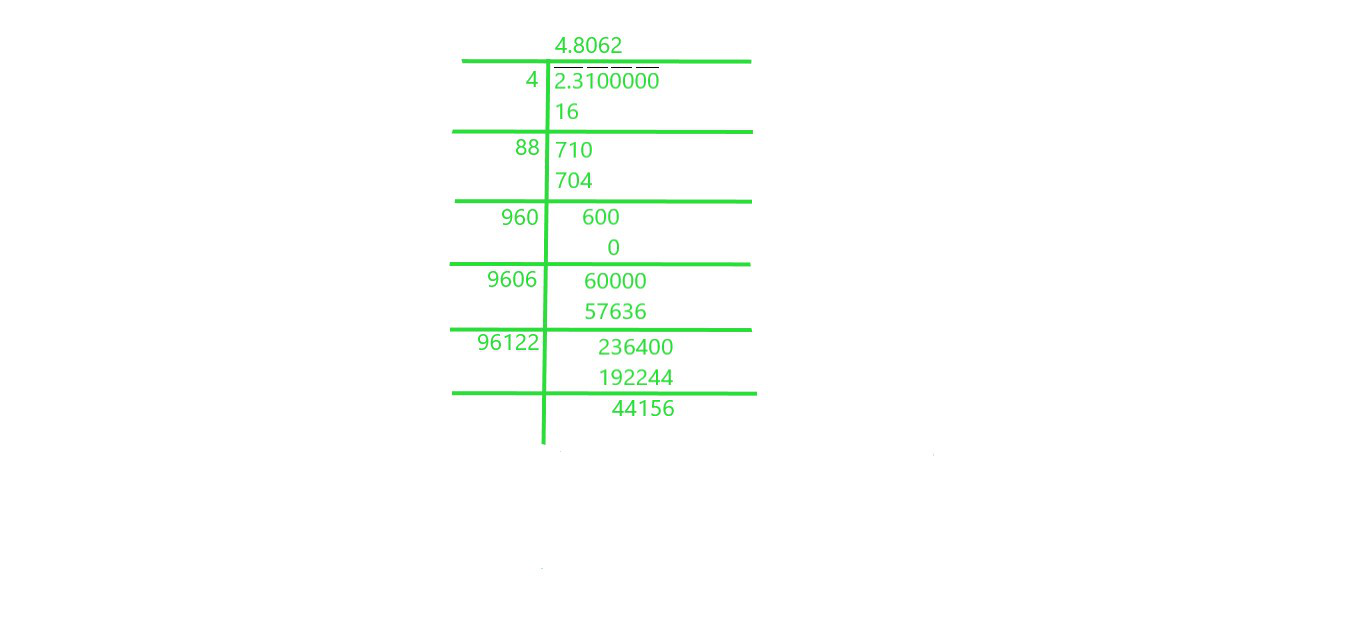Square root of 23.1 is 4.8062

### ix) 2.5

Solution: We will use Long Division Method to find square root of 2.5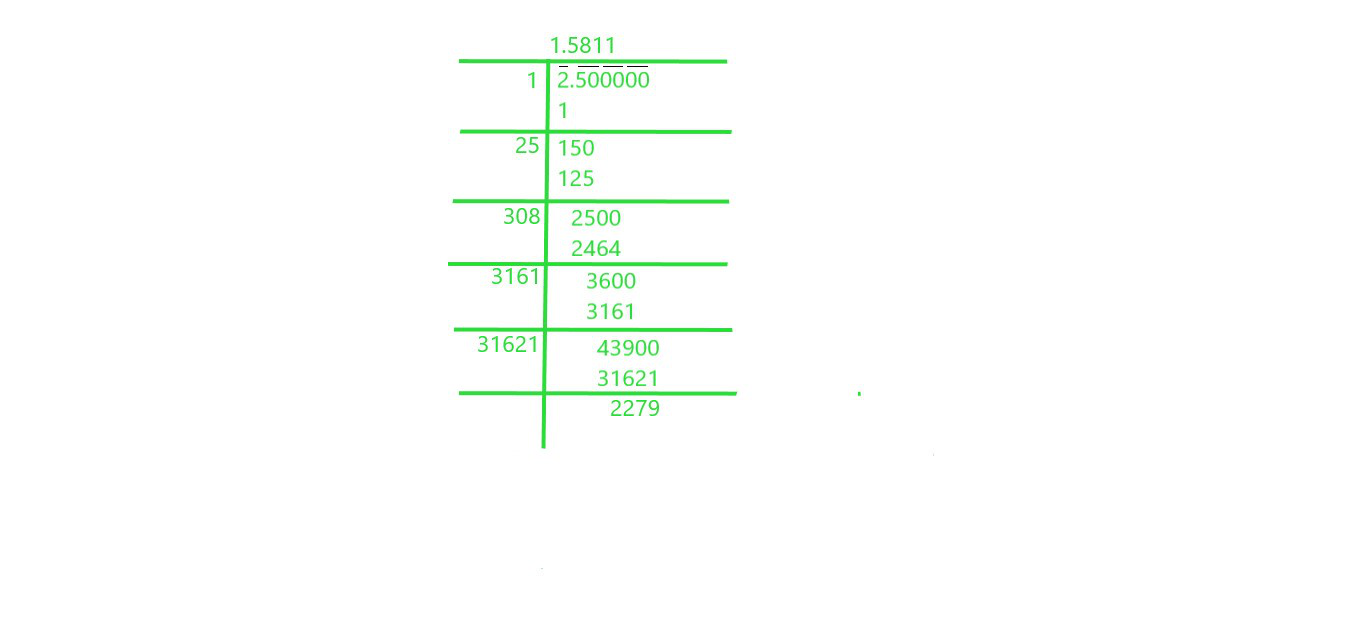Square root of 2.5 is 1.5811

### x) 237.615

Solution: We will use Long Division Method to find square root of 237.615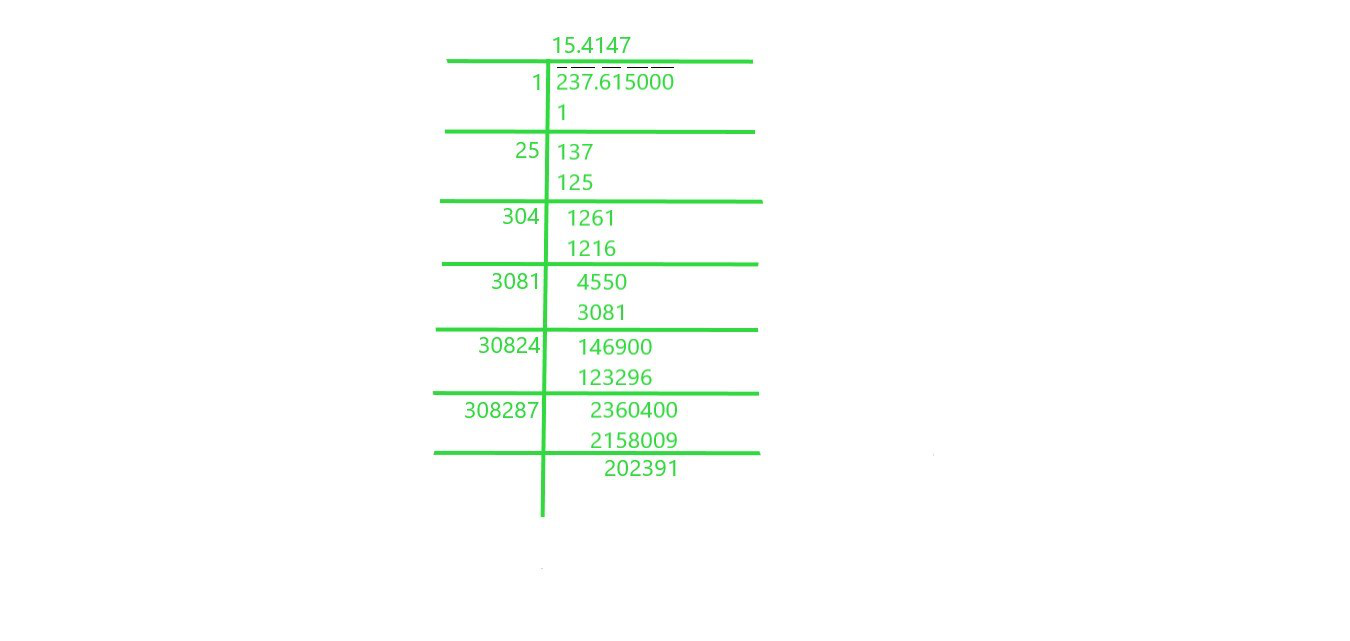Square root of 237.615 is 15.4147

### xi) 15.3215

Solution: We will use Long Division Method to find square root of 15.3215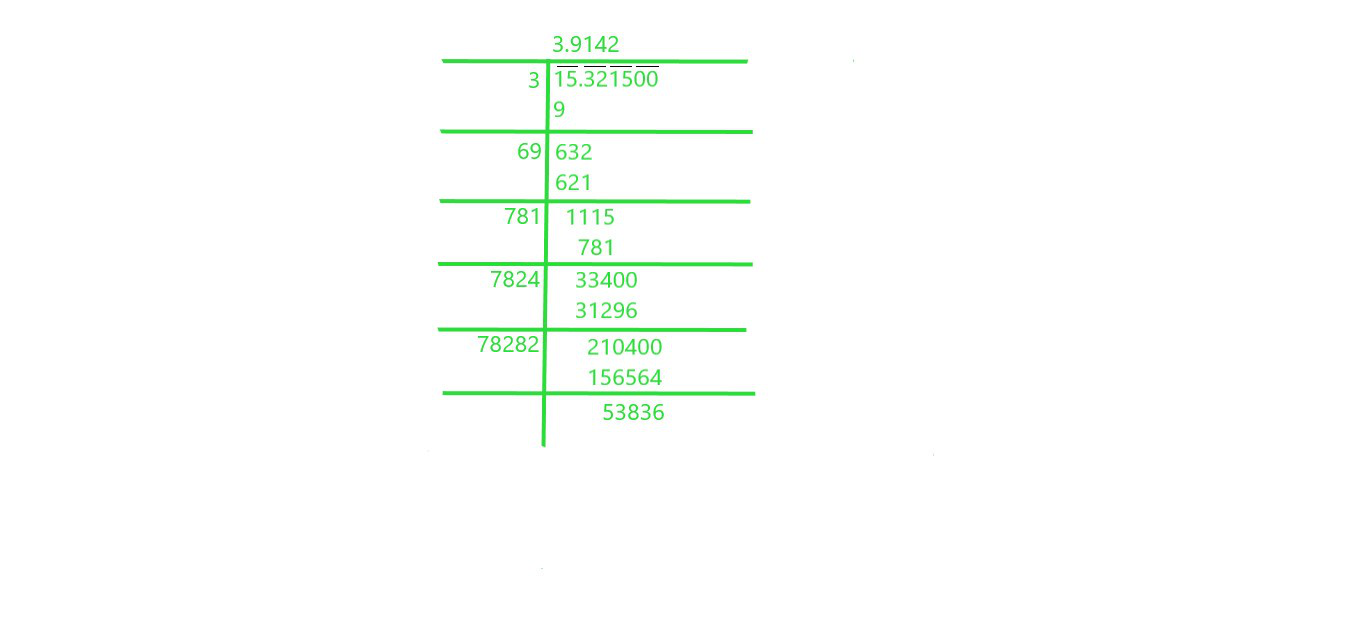Square root of 15.3215 is 3.9142

### xii) 0.9

Solution: We will use Long Division Method to find square root of 0.9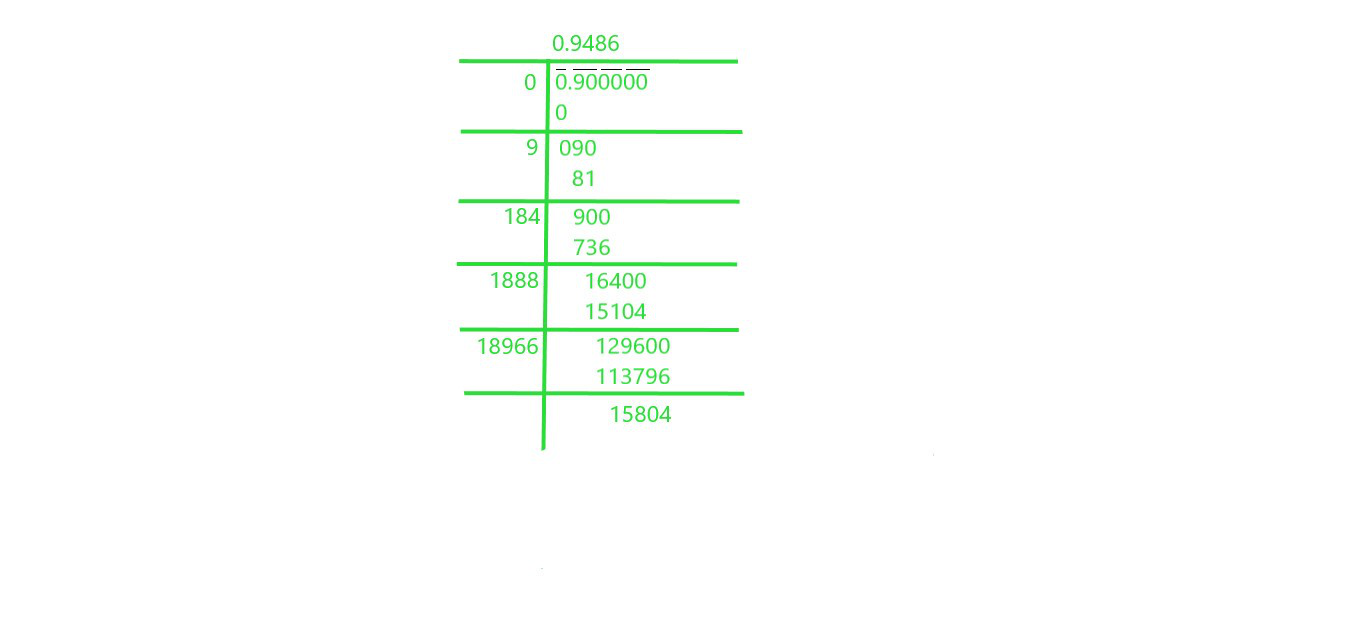Square root of 0.9 is 0.9486

### xiii) 0.1

Solution: We will use Long Division Method to find square root of 0.1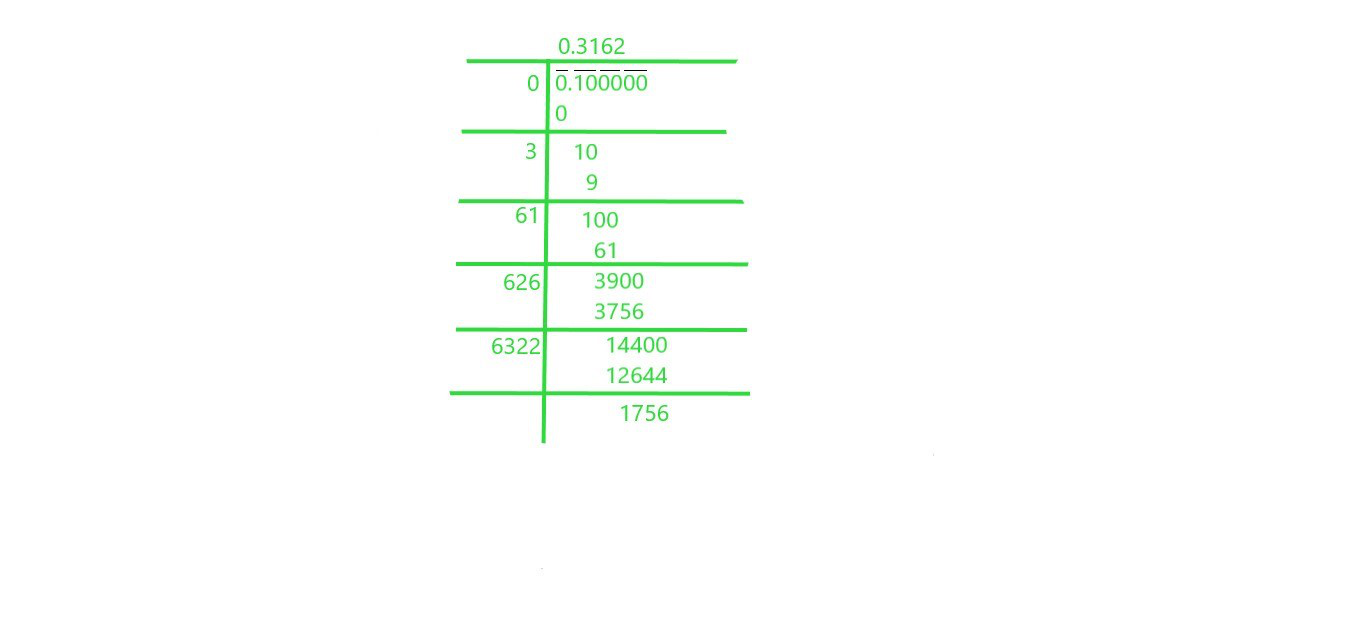Square root of 0.1 is 0.3162

### xiv) 0.016

Solution: We will use Long Division Method to find square root of 0.016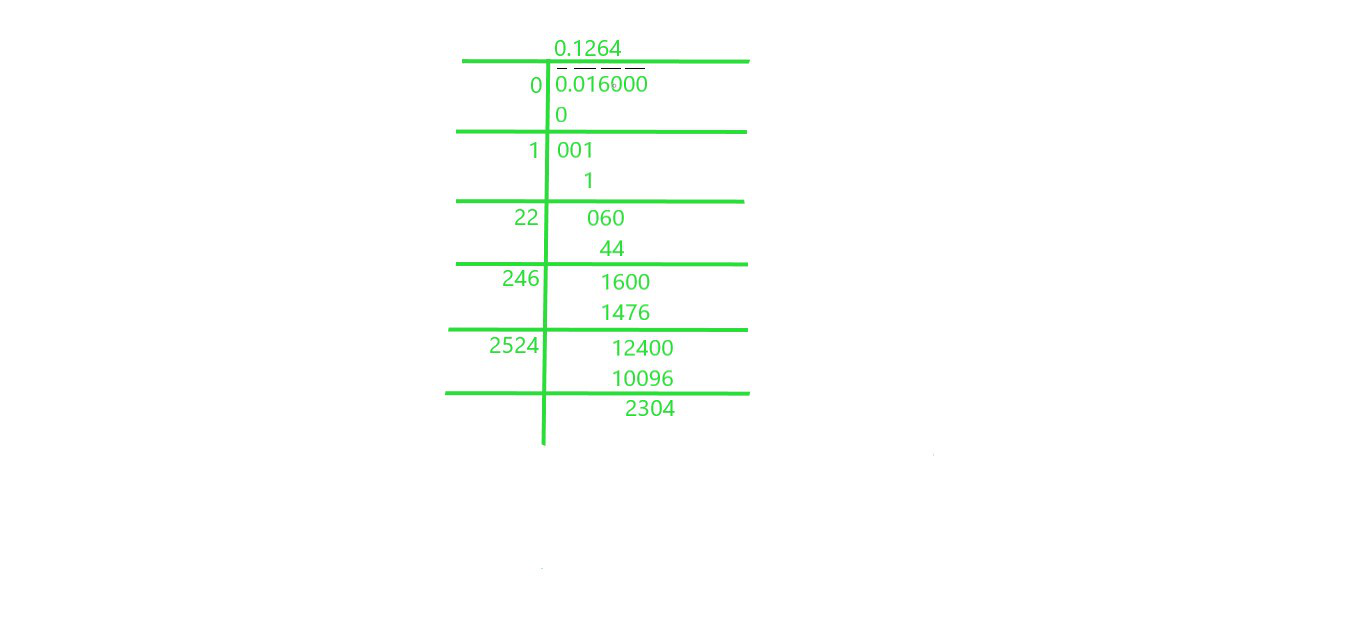Square root of 0.016 is 0.1264

### xv) 0.00064

Solution: We will use Long Division Method to find square root of 0.00064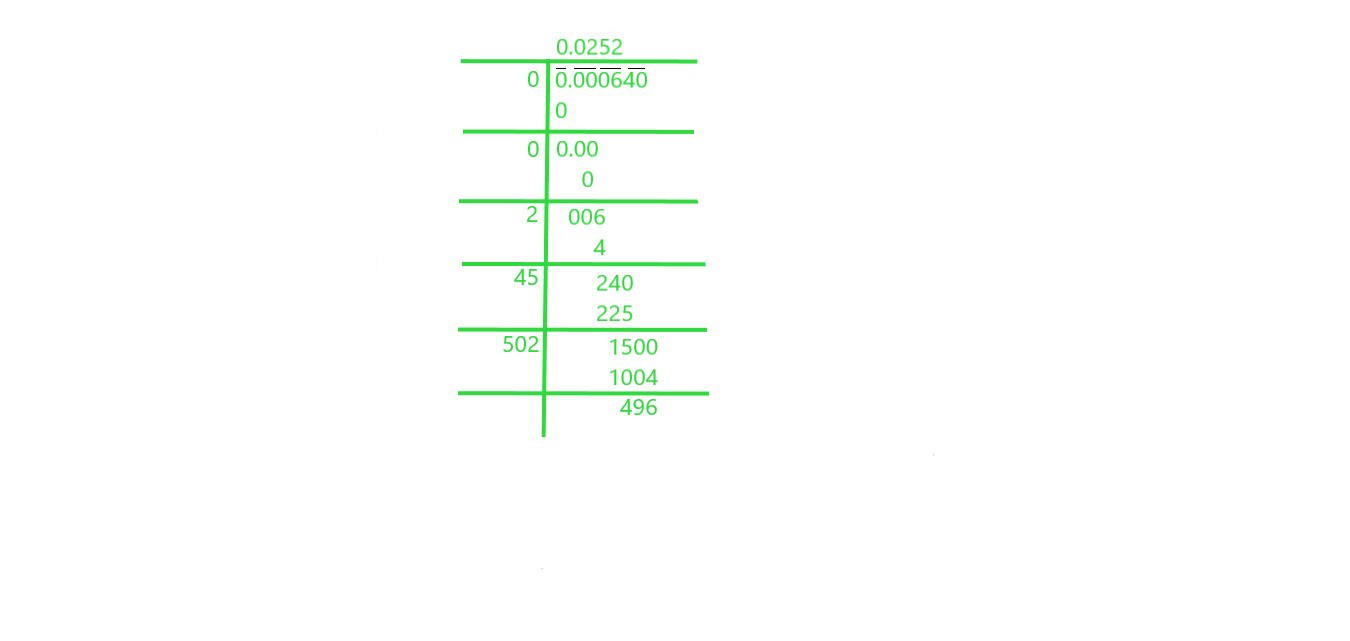Square root of 0.00064 is 0.0252

### xvi) 0.019

Solution: We will use Long Division Method to find square root of 0.019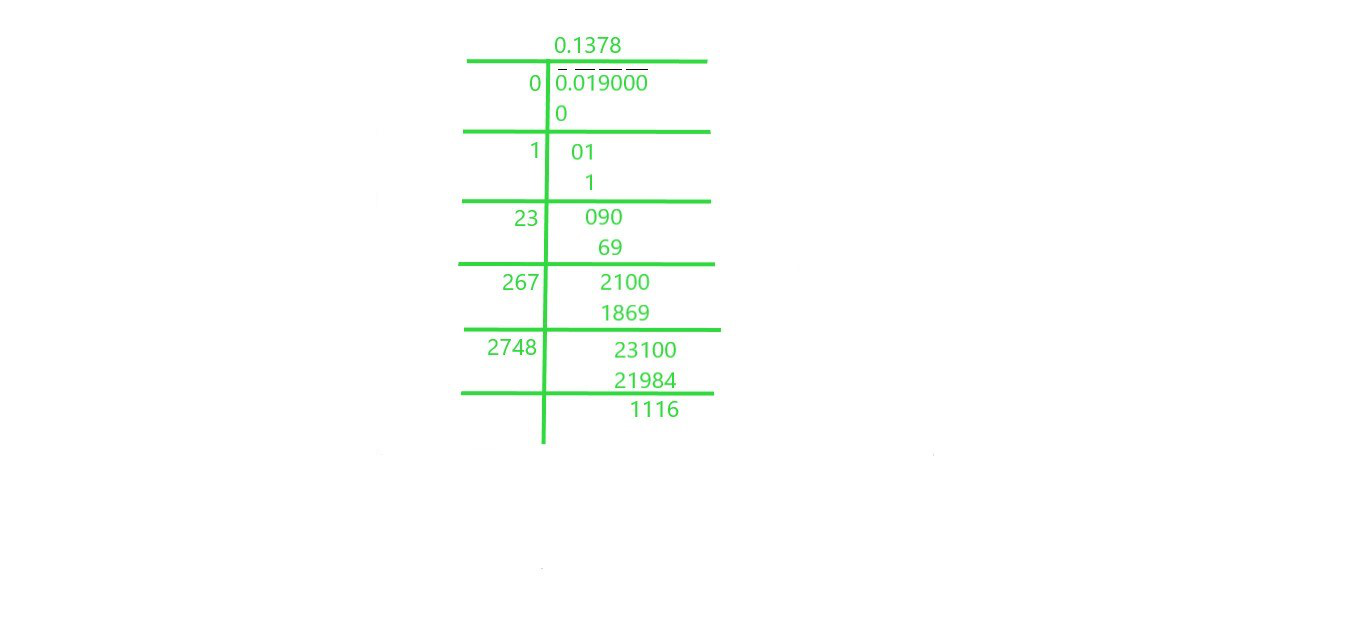Square root of 0.019 is 0.1378

### xvii) 7/8

Solution:  We will use Long Division Method to find square root of 7/8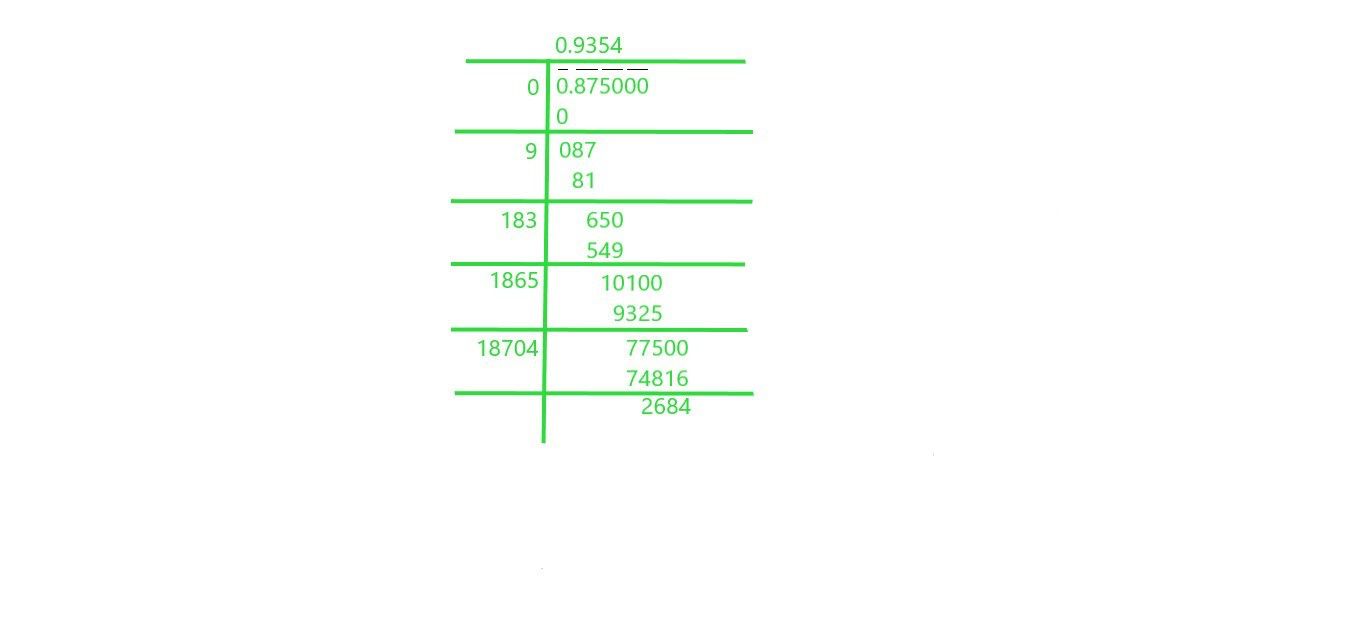Square root of 7/8 is 0.9354

### xviii) 5/12

Solution: We will use Long Division Method to find square root of 5/12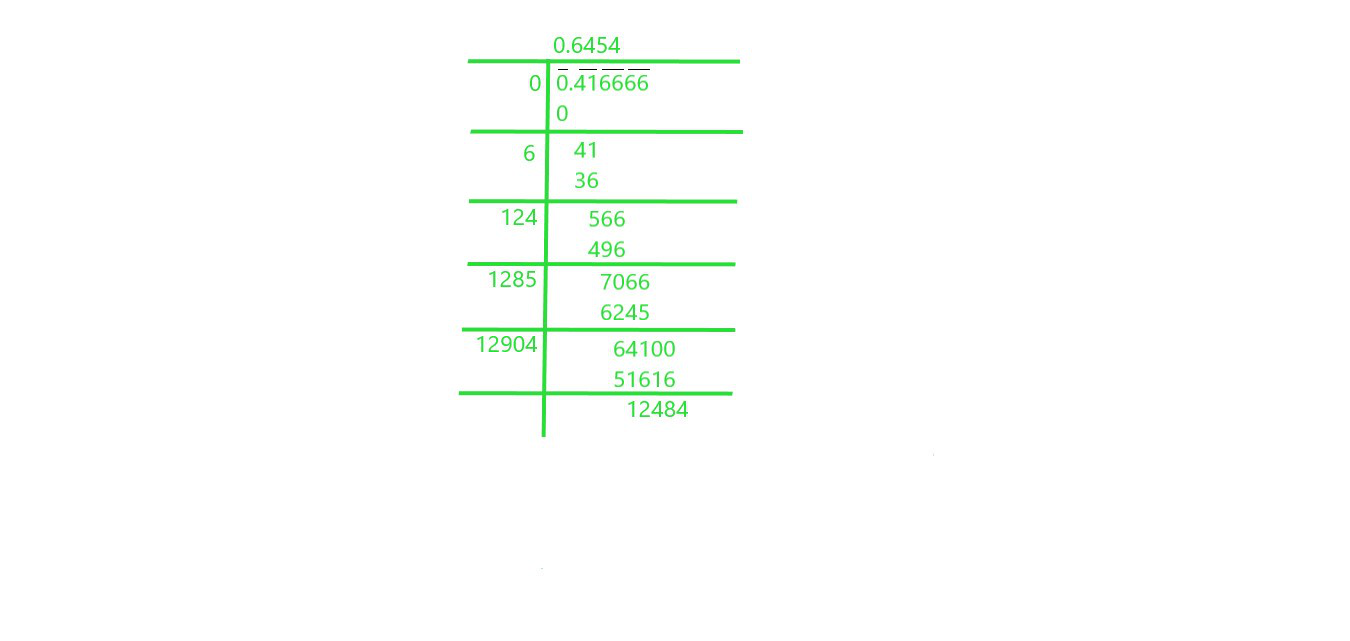Square root of 5/12 is 0.6454

### xix) 2 ½

Solution: We will use Long Division Method to find square root of 2 ½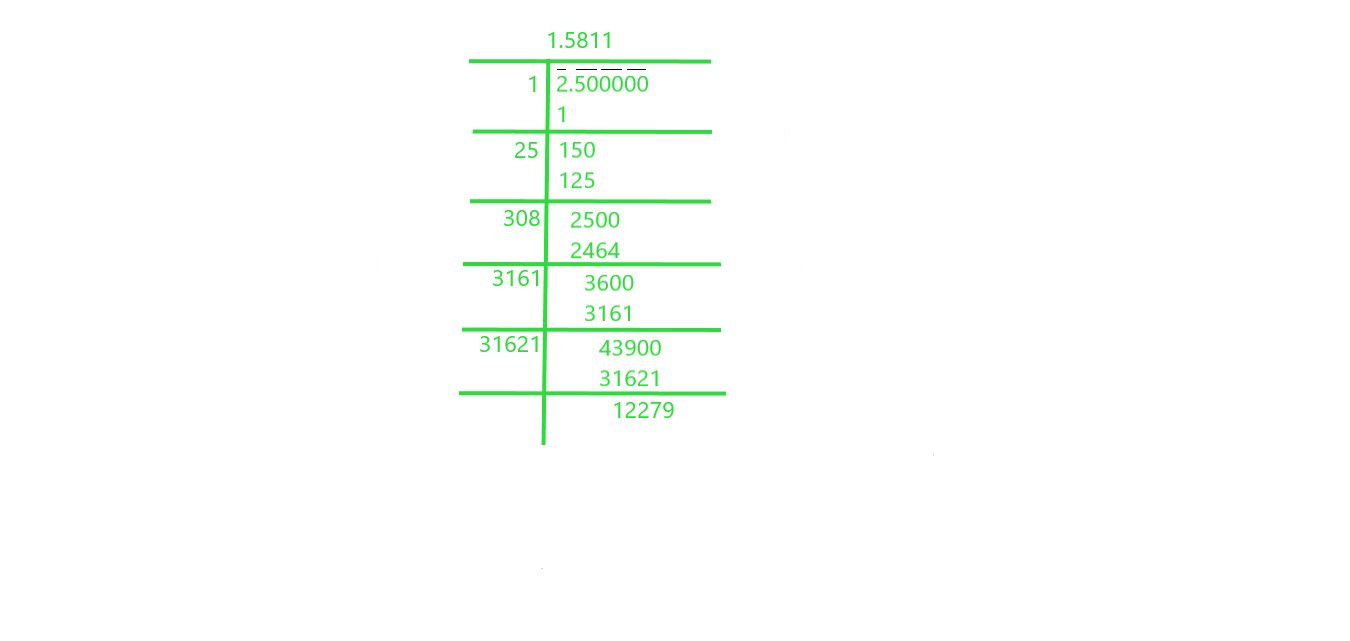Square root of 2 ½ is 1.5811

### xx) 287 5⁄8

Solution: We will use Long Division Method to find square root of 287 5⁄8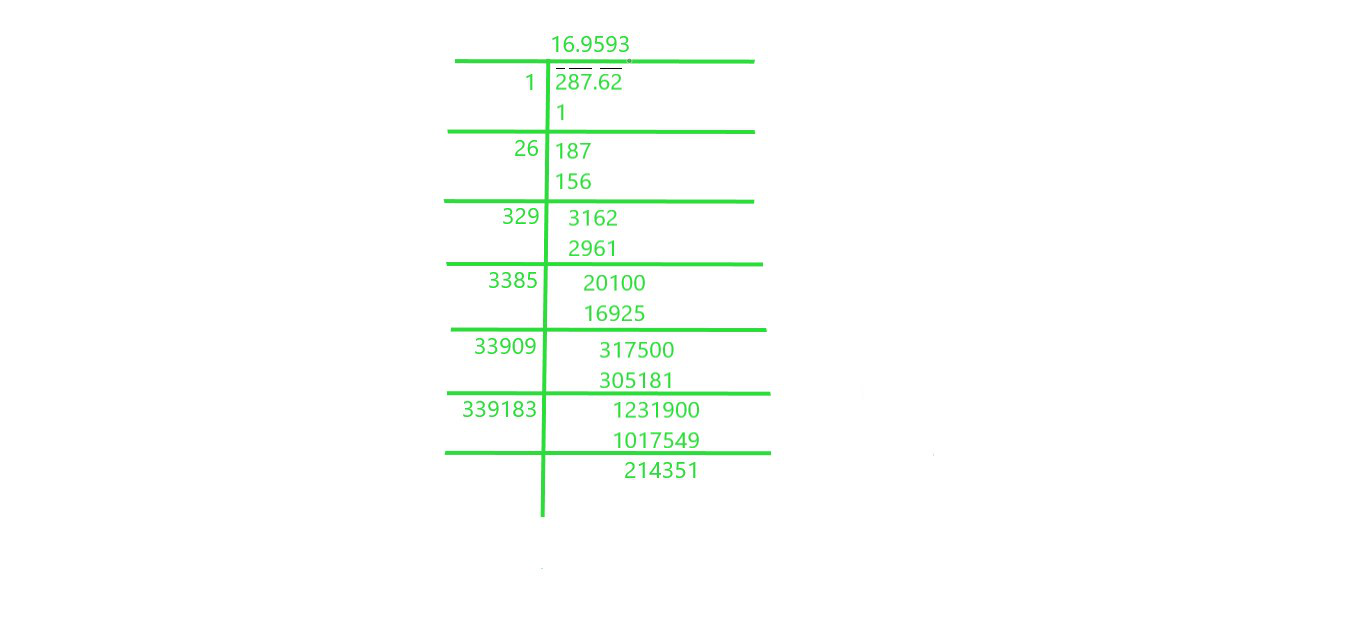Square root of 287 5⁄8 is 16.9593

### Question 2. Find the square root of 12.0068 correct to four decimal places.

Solution: We will use Long Division Method to find square root of 12.0068 correct to four decimal place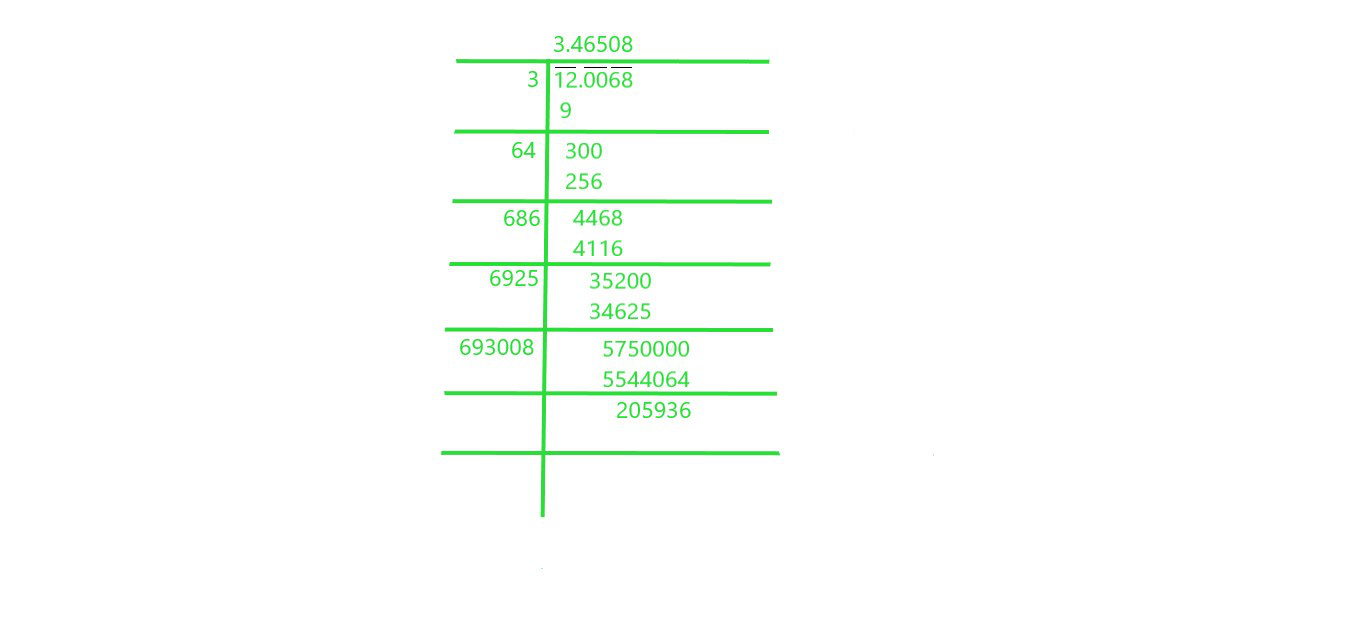The square root of 12.0068 correct to four decimal place is 3.46508

### Question 3. Find the square root of 11 correct to five decimal places.

Solution: We will use Long Division Method to find square root of 11 up to five decimal place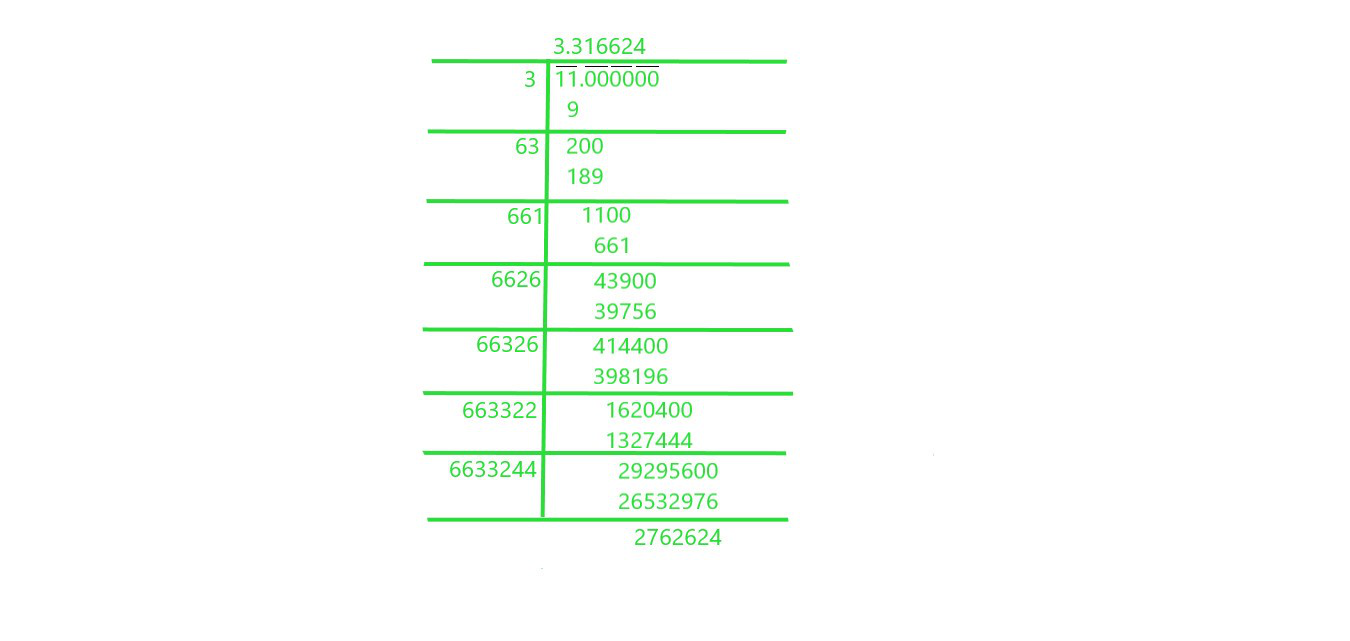The square root of 11 correct to five decimal place is 3.316624

### Question 4. Give that: √2 = 1.414, √3 = 1.732, √5 = 2.236 and √7 = 2.646, Evaluate each of the following :

(i) √(144/7)    (ii) √(2500/3)

Solution:

(i) √(144/7)

We can write √144 as √12×12 and we will calculate square root of √7

= 2.646 = √(12×12)/ √7

= 12/ 2.646 = 4.535

(ii) √(2500/3)

We will find factor of √2500 = √2x2x5x5x5x5, which is equal to 50 and we will calculate square root of √3

which is equal to 1.732 = 50/1.732 = 28.867

### Question 5. Given that √2 = 1.414, √3 = 1.732, √5 = 2.236 and √7 = 2.646 find the square roots of the following :

(i) 196/75     (ii) 400/63     (iii) 150/7     (iv) 256/5    (v) 27/50

Solution:

(i) 196/75

We have to calculate square root of √(196/75) , we can write it as √(196) / √(75)

Now find the factors of both numerator and denominator, we can write it as:

= √(14×14) / √(3x5x5) = 14/5√3

5√3 is equal to 5×1.732

= 8.66 = 1.617

(ii) 400/63

We have to calculate square root of √(400/63), we can write it as √(400)/√(63)

Now find the factors of both numerator and denominator, we can write it as

= √(20×20)/√(3x3x7)

= 20 / 3√7

3√7 is equal to 3×2.646

= 7.938

= 2.520

(iii) 150/7

We have to calculate square root of √(150/7), we can write it as √(150) / √(7)

Now find the factors of both numerator and denominator, we can write it as:

= √(3x5x5x2)/√(7) = 5x√3x√2 / √7

5x√3x√2 is equal to 5 x 1.732 x 1.414

= 12.245

= 12.245 / 2.646

= 4.628

(iv) 256/5

We have to calculate square root of √(256/5), we can write it as √(256) / √(5)

Now find the factors of both numerator and denominator, we can write it as:

= √(16×16) / √(5)

= 16 / √5

√5 is equal to 2.236

= 7.155

(v) 27/50

We have to calculate square root of √(27/50), we can write it as √(27) / √(50)

Now find the factors of both numerator and denominator, we can write it as:

= √(3x3x3) / √(2x5x5) = 3√3 / 5√2

3√3 is equal to 5.196 and 5√2 = 7.07

= 0.735

My Personal Notes arrow_drop_up